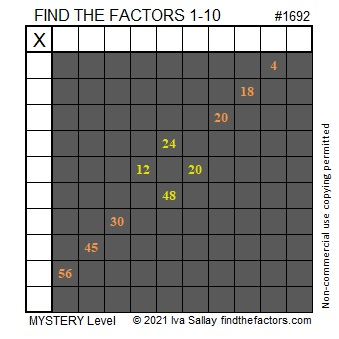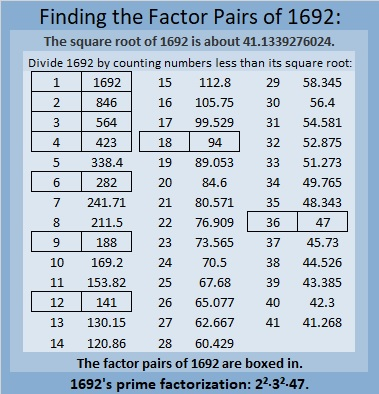# 1692 A Pilgrim’s Belt to Unbuckle

### Today’s Puzzle:

The logic needed to unbuckle this Pilgrim’s belt puzzle has several interesting twists and turns in it. Even adults will find it a challenge. Guessing and checking will only frustrate you. Use logic to write the numbers from 1 to 10 in both the first column and the top row so that those numbers and the given clues work like a multiplication table.Here’s the same puzzle if you want to print it using less ink.### Factors of 1692:

• 1692 is a composite number.
• Prime factorization: 1692 = 2 × 2 × 3 × 3 × 47, which can be written 1692 = 2² × 3² × 47.
• 1692 has at least one exponent greater than 1 in its prime factorization so √1692 can be simplified. Taking the factor pair from the factor pair table below with the largest square number factor, we get √1692 = (√36)(√47) = 6√47.
• The exponents in the prime factorization are 2, 2, and 1. Adding one to each exponent and multiplying we get (2 + 1)(2 + 1)(1 + 1) = 3 × 3 × 2 = 18. Therefore 1692 has exactly 18 factors.
• The factors of 1692 are outlined with their factor pair partners in the graphic below.
• 1568 is a composite number.### More About the Number 1692:

1692 is the difference of two squares in three different ways:
424² – 422² = 1692,
144² – 138² = 1692, and
56² – 38² = 1692.

The square of 1692 looks a little interesting:
1692² = 2862864.